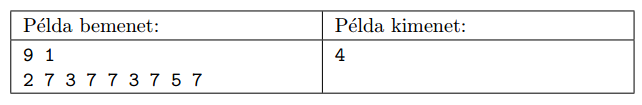Mathematical and Physical Journal
for High Schools
Issued by the MATFUND Foundation
 Already signed up? New to KöMaL?

#Problem S. 92. (October 2014)

S. 92. We are given $\displaystyle N$ ($\displaystyle 1\le N\le 2\;000\;000$) not necessarily distinct integers, each between 1 and 1000000000, in a sequence. Select $\displaystyle K$ ($\displaystyle 1\le K\le N$, $\displaystyle K$ being a given number) different integers from the sequence such that by deleting the selected numbers and all their copies from the sequence, the remaining sequence contains a longest connected subsequence of identical elements. Your program should read the values of $\displaystyle N$ and $\displaystyle K$ from the first line of the standard input, then the members of the sequence from the following line. The program should write the length of the longest possible subsequence of the above type to the first line of the standard output.

In the example, Példa bemenet'' is a sample input, and kimenet'' is the corresponding output. An explanation of the example is the following. By deleting all the 3s, the remaining sequence is 2 7 7 7 7 5 7, and here the four consecutive 7s form the longest identical subsequence. If we deleted a different number, we could not get a longer connected subsequence consisting of the same numbers in the remaining sequence.Scoring and bounds. You can obtain 1 point for a brief and proper documentation clearly describing your solution. Nine further points can be obtained provided that your program solves any arbitrary valid input within 1 second of running time.

The source code (s92.pas, s92.cpp, ...) without the .exe or any other auxiliary files generated by the compiler but with a short documentation (s92.txt, s92.pdf, ...), also describing which developer environment to use for compiling the source, should be submitted in a compressed file s92.zip.

(10 pont)

Deadline expired on November 10, 2014.

### Statistics:

 12 students sent a solution. 10 points: Csenger Géza, Gáspár Attila, Németh 123 Balázs, Weisz Ambrus. 9 points: Zarándy Álmos. 6 points: 1 student. 4 points: 1 student. 3 points: 2 students. 1 point: 3 students.

Problems in Information Technology of KöMaL, October 2014